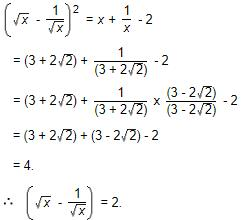Courses

# Power And Index MCQ 2

## 15 Questions MCQ Test Quantitative Techniques for CLAT | Power And Index MCQ 2

Description
This mock test of Power And Index MCQ 2 for Quant helps you for every Quant entrance exam. This contains 15 Multiple Choice Questions for Quant Power And Index MCQ 2 (mcq) to study with solutions a complete question bank. The solved questions answers in this Power And Index MCQ 2 quiz give you a good mix of easy questions and tough questions. Quant students definitely take this Power And Index MCQ 2 exercise for a better result in the exam. You can find other Power And Index MCQ 2 extra questions, long questions & short questions for Quant on EduRev as well by searching above.
QUESTION: 1

###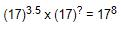Solution:QUESTION: 2

### If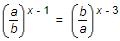, then the value of x is:

Solution: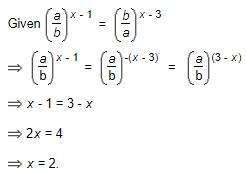QUESTION: 3

### Given that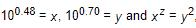then the value of z is close to

Solution: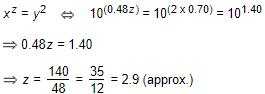QUESTION: 4

​If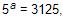then the value of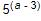is:

Solution: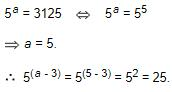QUESTION: 5

​If 3(x - y) = 27 and 3(x + y) = 243, then x is equal to:

Solution: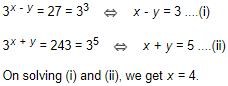QUESTION: 6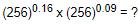Solution: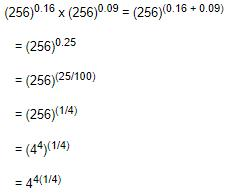= 41

= 4

QUESTION: 7

The value of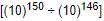Solution: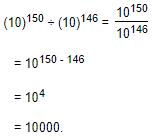QUESTION: 8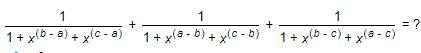Solution: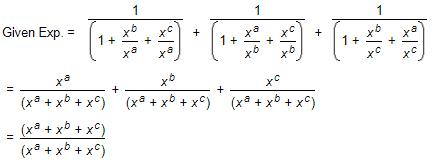= 1

QUESTION: 9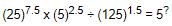Solution: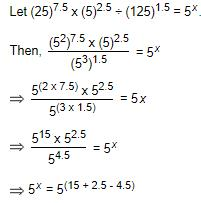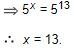QUESTION: 10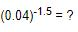Solution: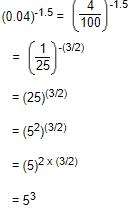= 125

QUESTION: 11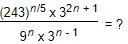Solution:

Given Expression =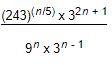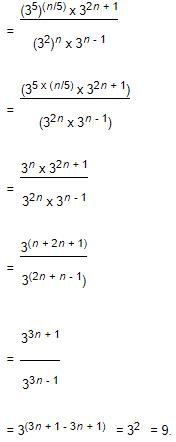QUESTION: 12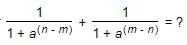Solution: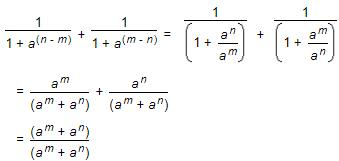= 1

QUESTION: 13

​If m and n are whole numbers such that mn = 121, the value of (m - 1)n + 1 is:

Solution: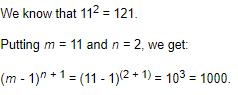QUESTION: 14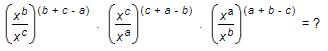Solution:

Given Exp.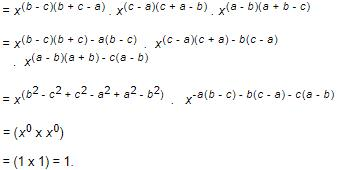QUESTION: 15

If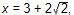then the value of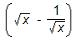is:

Solution: